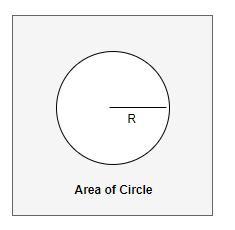# Area of Circle calculator

Note
• Enter the values of Either Radius,Diameter,Area or Circumference
• Click on the calculate button to get the value of empty

Formula And concept used
if r is radius of the Circle,then
$\text {Diameter} = 2r$
$\text {Area of Circle}= \pi r^2$
$\text{circumference}= 2 \pi r$

## What is SphereCircle is a two dimensional geometricial shape.It is defined mathematically as the set of points that are all at the same distance r from a given point in a two dimensional space..
The distance r is called the Radius of the Circle.
Diameter of the Circle is two times radius of the Circle
$D= 2r$
Area of Circle is given by the formula
$\text{Area}= \pi r^2$
Circumference of circle is given by
$\text{circumference}= 2 \pi r$

Example of Few questions where you can use this Area of Circle Calculator
Question 1
Find the Area and Circumference of a Circle whose radius is
i. 3 cm
ii. 10 cm
Solution
(i) r=3 cm, A=? Area of Circle is given by the formula
$A= \pi r^2= 3.14 \times 3^2 =28.26 \ cm^2$
Circumference of circle is given by
$C= 2 \pi r =2 \times 3.14 \times 3 =18.84 \ cm$

(i) r=10 cm, A=? Area of Circle is given by the formula
$A= \pi r^2= 3.14 \times 10^2 =314 \ cm^2$
Circumference of circle is given by
$C= 2 \pi r =2 \times 3.14 \times 10 =62.8 \ cm$

Question 2
Find the radius,Diameter and Circumference of the circle whose Area is 628 cm2
Solution
A=628 cm2, D=? ,r=?, C=? Area of Circle is given by the formula
$A= \pi r^2$
$r= \sqrt { \frac {A}{\pi}} = \sqrt { \frac {628}{3.14}}= 14.14 \ cm$
Now
Circumference of circle is given by
$C= 2 \pi r =2 \times 3.14 \times 14.14 =88.81 \ cm$
Diameter is given by
D= 2r = 28.18 cm

Question 3
Find the radius,Diameter and Area of the circle whose Circumference is 12.56 cm
Solution
A=?, D=? ,r=?, C=12.56 cm
Circumference of circle is given by
$C= 2 \pi r$
$r= \frac {C}{2 \pi} = \frac {12.56}{2 \times 3.14} =2 \ cm$ Area of Circle is given by the formula
$A= \pi r^2 = 3.14 \times 2^=12.56 \ cm^2$
Diameter is given by
D= 2r = 4 cm

## How Calculator works

• if r is radius of the Circle,then calculation done as below formula
$\text {Diameter} = 2r$
$\text {Area}=\pi r^2$
$\text{circumference}= 2 \pi r$
• if Area of the Circle is given,then calculation done as below formula
$\text {radius} = \sqrt { \frac {A}{\pi}}$
$\text {Diameter}=2r$
$\text{circumference}= 2 \pi r$
• if circumference of the Circle is given,then calculation done as below formula
$\text {radius} = \frac {C}{2\pi}$
$\text {Diameter}=2r$
$\text {Area}=\pi r^2$
• if Diameter of the Circle is given,then calculation done as below formula
$\text {radius} = \frac {D}{2}$
$\text{circumference}= 2 \pi r$
$\text {Area}=\pi r^2$